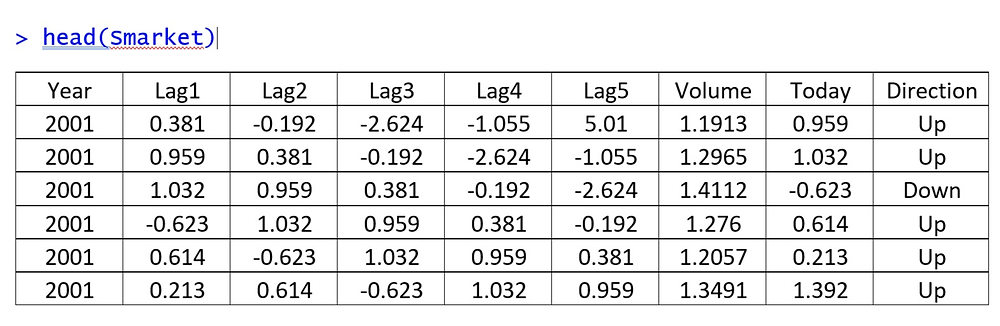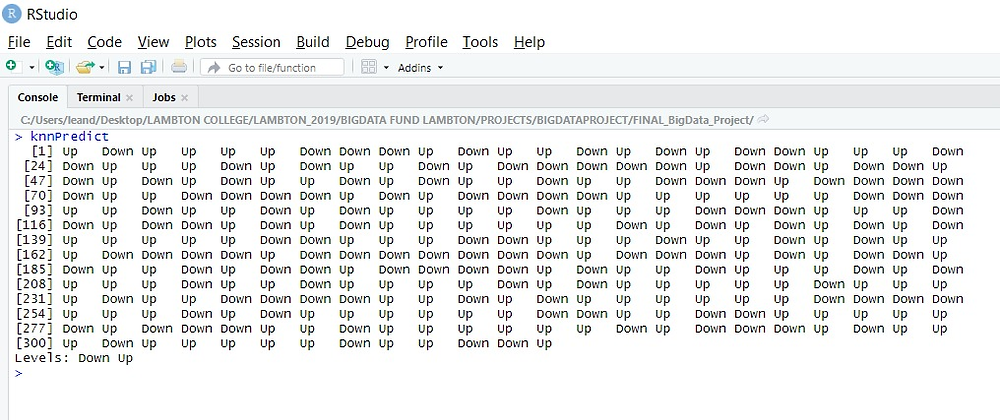Search

# Stock Market Prediction via Machine Learning## Summary

The purpose of presenting another machine learning tool and its applicability, we will present an approach to the application of an algorithm called KNN, which aims to perform binaries classification forecasts within the universe of the Stock Exchange. To this end, we will use the R programming language, which through the reading of a historical database of the Stock Exchange, will develop a predictive model and its accuracy.

## Introduction

Currently, there has been a growing use of machine learning tools to support decision-making in the business world and especially in the Stock Exchange, these tools have been increasingly used and their applicability has extended to the universe of different types of business, presenting itself as a strong tool to support decision making. Problem Description Predicting the outcome of the S&P index (The Standard & Poor's 500) American stock market index (NYSE or NASDAQ)

## Lifecycle### Format

A data frame with 1250 observations on the following 9 variables.

- Year

The year that the observation was recorded

- Lag1

Percentage return for previous day

- Lag2

Percentage return for 2 days previous

- Lag3

Percentage return for 3 days previous

- Lag4

Percentage return for 4 days previous

- Lag5

Percentage return for 5 days previous

- Volume

- Today

Percentage return for today

- Direction

A factor with levels Down and Up indicating whether the market had a positive or negative return on a given day

### Source

Raw values of the S&P 500 were obtained from Yahoo Finance and then converted to percentages and lagged.

Balancing the Dataset

Checking the original and partitional data distribution, we have to keep the data balanced, if there are more cases than another, the model will have a vies because it could learn more about the error.

Therefore, for the case below, the data is balanced with the number of cases down and up

if there was a lot of difference between the number of cases, we would have to do a class balance.

```> prop.table(table(Smarket\$Direction)) * 100

Down Up
48.16 51.84
> prop.table(table(data_train\$Direction)) * 100

Down Up
48.18763 51.81237 ```

### Data Normalization Process

KNN Classification with R - Data normalization(Center e Scale) putting all the data on the same scale.

The scale transformation calculates the standard deviation for an attribute and divides

each value by this standard deviation.

The "center" transformation calculates the average of an attribute and subtracts it from each value.

```
#Function of Normalization
scale.features <- function(df, variables){
for (variable in variables){
df[[variable]] <- scale(df[[variable]], center = T, scale = T)
}
return(df)
}```
```#Removing the target variable from the training and test data
numeric.vars_train <- colnames(trainX <- data_train[,names(data_train) != "Direction"])
numeric.vars_test <- colnames(testX <- data_test[,names(data_test) != "Direction"])
# Applying normalization to predictor variables of training and testing
data_train_scaled <- scale.features(data_train, numeric.vars_train)
data_test_scaled <- scale.features(data_test, numeric.vars_test)```

### Creation of the Model

```knn_v1 <- train(Direction ~ .,
data = data_train_scaled,
method = "knn",
trControl = ctrl,
# preProcess = c("center","scale"), # Different way for normalization model
tuneLength = 20)```

### KNN Classification in R - Model Evaluation```# Number of Neighbors x Accuracy
plot(knn_v1)```### Predictions

```# Making predictions
knnPredict <- predict(knn_v1, newdata = data_test_scaled)
knnPredict``````# Creating a Confusion Matrix
confusionMatrix(knnPredict, data_test\$Direction)```### KNN Classification in R - Applying Other Metrics

```# Control file
ctrl <- trainControl(method = "repeatedcv",
repeats = 3,
classProbs = TRUE,
summaryFunction = twoClassSummary)```
```# Training the model
knn_v2 <- train(Direction ~ .,
data = data_train_scaled,
method = "knn",
trControl = ctrl,
metric = "ROC",
tuneLength = 20)`````` # Number of Neighbors x Accuracy
> plot(knn_v2, print.thres = 0.5, type="S")```### Predictions

```# Making predictions
knnPredict <- predict(knn_v2, newdata = data_test_scaled)
knnPredict``````# Creating a Confusion Matrix
confusionMatrix(knnPredict, data_test\$Direction)```### Forecast with New Data

```# Forecasts with new data
# Preparing input data
Year = c(2006, 2007, 2008)
Lag1 = c(1.30, 0.09, -0.654)
Lag2 = c(1.483, -0.198, 0.589)
Lag3 = c(-0.345, 0.029, 0.690)
Lag4 = c(1.398, 0.104, 1.483)
Lag5 = c(0.214, 0.105, 0.589)
Volume = c(1.36890, 1.09876, 1.231233)
Today = c(0.289, -0.497, 1.649)

new_data = data.frame(Year, Lag1, Lag2, Lag3, Lag4, Lag5, Volume, Today)	```### Normalizing the Data

```#Extracting variable names
names_variables <- colnames(new_data)
names_variables```
```# Applying the function
new_data_scaled <- scale.features(new_data, names_variables)
new_data_scaled
str(new_data_scaled)
class(new_data_scaled)```

### Predictions

```# Making Predictions
knnPredict <- predict(knn_v2, newdata = new_data_scaled)
cat(sprintf("\n Predictio of \"%s\" é \"%s\"\n", new_data\$Year, knnPredict))```
• Prediction of "2006" is "Down"

• Prediction of "2007" is "Down"

• Prediction of "2008" is "Up"

### Final Conclusion

So, when we compare the accuracy results between KNN_v1 and KNN_v2, we conclude that we KNN_v2 had a better accuracy = 88.78%.

We can improve the accuracy, creating different scenarios.

After all these steps above, with the model selected, we only import the dataset with the same format into R and them we just need to apply and publish the model will return the prediction for support decision investment in the stock market.

Although, we can display any stock market information in real time to drive decision, using Power Bi visualization, as we have demonstrated in other articles.

These innovations technologies tolls together can support your business to drive decision for the next level.

### Benefits of Stock Market Prediction via Machine Learning.

• Minimize the Investment Risk.

• Better Return of Investment.

12 views